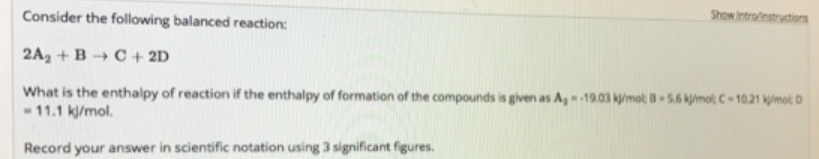# Problem: Consider the following balanced reaction: 2A2 + B → C + 2D What is the enthalpy of reaction if the enthalpy of formation of the compounds is given as A2 = 19.03  kJ/mol; B = 5.6 kJ/mol; C = 10.21 kJ/mol; D = 11.1 kJ/mol. Record your answer in scientific notation using 3 significant figures.

###### FREE Expert Solution
90% (359 ratings)###### Problem Details

Consider the following balanced reaction:

2A2 + B → C + 2D

What is the enthalpy of reaction if the enthalpy of formation of the compounds is given as A2 = 19.03  kJ/mol; B = 5.6 kJ/mol; C = 10.21 kJ/mol; D = 11.1 kJ/mol.